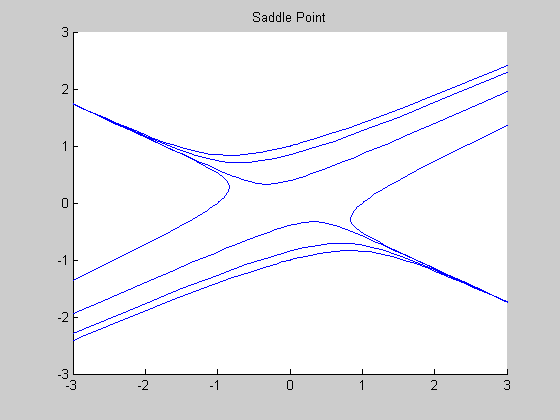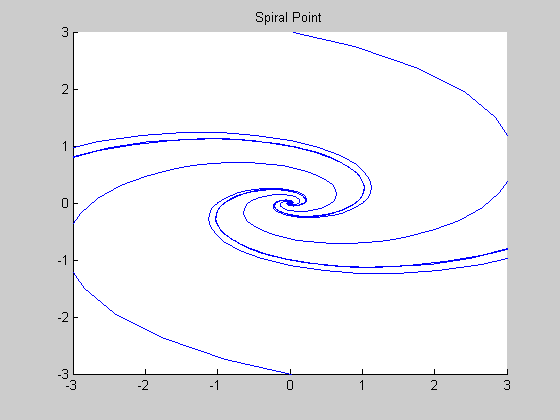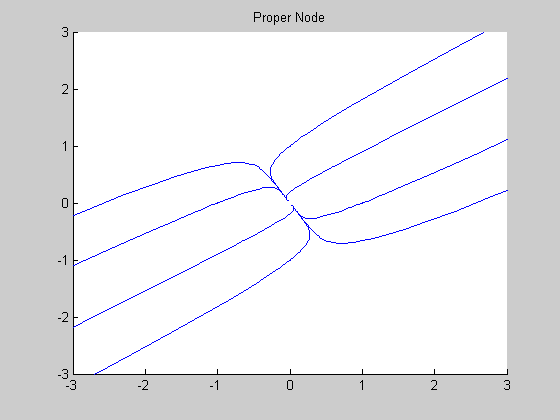Examples of Homogeneous Linear Systems

Contents

A = [1 3; 1 1]
eig(A)
A =

1     3
1     1

ans =

2.7321
-0.7321

Phase Portrait: let's take initial conditions spaced around a circle

figure, hold on
for j=1:8
[t, y] = ode45(@(t, y) A*y, [0, 4], [cos(2*j*pi/8), sin(2*j*pi/8)]);
plot(y(:,1), y(:, 2))
[t, y] = ode45(@(t, y) A*y, [0, -4], [cos(2*j*pi/8), sin(2*j*pi/8)]);
plot(y(:,1), y(:, 2))
end
axis([-3,3,-3,3]), hold offCase of a Spiral Point

A = [1 -4; 1 1]
eig(A)
A =

1    -4
1     1

ans =

1.0000 + 2.0000i
1.0000 - 2.0000i

Phase Portrait: let's take initial conditions spaced around a circle

figure, hold on
for j=1:8
[t, y] = ode45(@(t, y) A*y, [0, 4], [cos(2*j*pi/8), sin(2*j*pi/8)]);
plot(y(:,1), y(:, 2))
[t, y] = ode45(@(t, y) A*y, [0, -4], [cos(2*j*pi/8), sin(2*j*pi/8)]);
plot(y(:,1), y(:, 2))
end
axis([-3,3,-3,3]), hold off
title 'Spiral Point'Case of a Proper Node

A = [2 1; 1 1]
eig(A)
A =

2     1
1     1

ans =

2.6180
0.3820

Phase Portrait: let's take initial conditions spaced around a circle

figure, hold on
for j=1:8
[t, y] = ode45(@(t, y) A*y, [0, 4], [cos(2*j*pi/8), sin(2*j*pi/8)]);
plot(y(:,1), y(:, 2))
[t, y] = ode45(@(t, y) A*y, [0, -4], [cos(2*j*pi/8), sin(2*j*pi/8)]);
plot(y(:,1), y(:, 2))
end
axis([-3,3,-3,3]), hold off
title 'Proper Node'